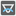• # AssertionError: All sides are equal

## Question related to missionThe Angles of a Triangle

Hi! well, this is my solution to the problem, but for some reason when I run it I get "AssertionError: All sides are equal". my problem is that the code working in pycharm and jupyter notebook, so I don't have a slight clue where is the problem. thanks for the help

```def checkio(a,b,c):
lf=[]
try:
if (a * a + b * b - c * c)==0:
lf.append(int(round(math.degrees(math.asin((a*1.0)/c)))))
lf.append(int(round(math.degrees(math.asin((b*1.0)/c)))))
lf.append(90)
return sorted(lf)
else:
lf.append(int(round(math.degrees(math.acos((a * a + b * b - c * c)/(2.0 * a * b))))))
lf.append(int(round(math.degrees(math.acos((a * a + b * b - c * c)/(2.0 * a * c))))))
lf.append(int(round(math.degrees(math.acos((a * a + b * b - c * c)/(2.0 * c * b))))))
return sorted(lf)
except:
return [0,0,0]
```6